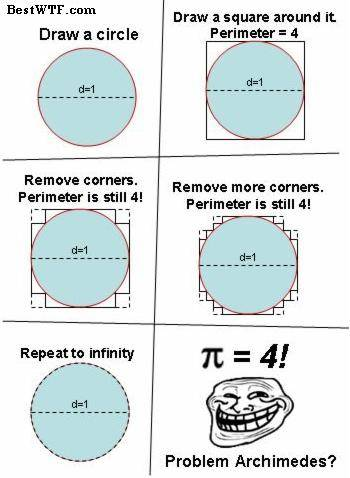# Finding Circle Circumference from Inscribed N-Sided Polygen

logan3
The perimeter P of a regular polygon of n sides inscribed in a circle of radius r is given by $P = 2nr sin (180^o / n)$.

I was curious whether it's possible to approximate the circumference of a circle by taking the limit as n goes to infinity of the above perimeter equation is some way?

Thank-you

## Answers and Replies

Gold Member
I would think it should be possible. As it stands, applying that limit would give you infinite*0.
Is there anyway you could express the same function in terms of other trig functions that would give you inf/inf or 0/0? Then you could potentially apply l'hopital's rule and get something useful.

logan3
$P = 2nr \sin (180^o / n) = \frac {2nr} {\csc (180^o / n)} = \frac {2nr} {\csc (180^o / n)}$
$\displaystyle \lim_{n\rightarrow \infty} {\frac {2nr} {\csc (180^o / n)}} = \frac {\infty} {\infty}$

L'Hôpital's rule:
$\displaystyle \lim_{n\rightarrow \infty} {\frac {f'(n)} {g'(n)}} = \displaystyle \lim_{n\rightarrow \infty} {\frac {2r} {-\csc (180^o / n) \cot (180^o / n)}}$

Sorry, this is about as far as I got right now. Also, I'm not sure where the $\pi$ is going to come in for the $C = 2\pi r$, though I assume it will have to come from the trig functions somehow.

$\lim_{n->\infty}\frac {sin(\frac{\pi}{n})}{\frac{\pi}{n}}=1$.
Therefore $\lim_{n->\infty}2nrsin(\frac{\pi}{n})=2\pi r$.

•acegikmoqsuwy
logan3
Where's the denominator of pi / n come from in the second expression?

Staff Emeritus
Homework Helper
It works in this case. But you need to be careful with polygonal approximation of lengths, it might not always give the right answers:•BiGyElLoWhAt
Gold Member
It works in this case. But you need to be careful with polygonal approximation of lengths, it might not always give the right answers:
Hehe. :D

Where's the denominator of pi / n come from in the second expression?
P=2nrsin(π/n)=2πr(sin(π/n)/(π/n))

logan3
I don't understand, sorry.

But it seems like my thoughts about this relationship were in the right direction.

Gold Member
Its just algebra that he did to get it into a new form. Multiply by pi/pi, and kick the n into the denominator of the denominator. Now you have sin (x)/x.